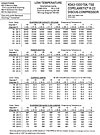Figure 1. Table-type compressor performance curve. (Courtesy of Copeland Corp.)
Compressor performance curves can provide the service technician with the Btu pumping rate (capacity), mass flow rates, operating amperage, and operating wattage, when the suction pressure and head pressure are known for the system. Compressor curves are available from the compressor manufacturer for each of their compressors. Compressor data comes in either graph or table type (see Figure 1).

This article will take a closer look at the table type data and concentrate on the current (amps) performance data.

Let’s say compressor data shown is a 10-horsepower, semi-hermetic, low-temperature, Discus model using R-22 as the refrigerant.

The first column in Figure 1 gives the rated conditions and specifications. The rest of the table gives capacity data, power (watts), current (amps), and evaporator mass flow rate (pounds per hour).

## EXAMPLE PROBLEM

Let’s assume this compressor is operating at 230 V and has an evaporator temperature of 0°F and a condensing temperature of 90°. Determine the correct current (amp) draw for this compressor using Figure 1.

Solution: The intersection of the 90° condensing temperature with a 0° evaporating temperature results in an amp draw of 31.6 amps (A). This data tells us that this compressor operating under these pressures should draw 31.6 A at 230 V. If the compressor were operating at 460 V, the amperage values in the table would have to be multiplied by one-half.

If the technician now actually measures the compressor amps with an ammeter at the compressor and finds the amps to be very close to the 31.6 they should be at these pressures (see Figure 1), the technician has a pretty good idea that the compressor is up to specifications. If, however, the technician measures the current draw of the compressor and finds that it is pulling only 24 A at these same conditions, there is a problem somewhere.

## CAUSES FOR LOW AMP

Here is a list of causes for a compressor pulling low amperage:

• Bad suction and/or discharge valve;

• Starved evaporator;

• Worn rings;

• Restricted liquid line or filter drier;

• Undercharged system;

• Weak motor; and

• Metering device starving evaporator.

The technician must now systematically check each of the above causes and find the one that is the cause for the low compressor amp draw.

Also note that as the condensing temperature decreases, so do the amps. This is assuming a constant evaporating temperature. Also, as the evaporating temperature decreases, so do the amps. This is assuming a constant condensing pressure. Decreased condensing temperatures mean lower condensing pressures. The compressor’s piston now operates with less pressure against it as it compresses the suction gases. This in turn is less stress on the motor and less amperage draws.

As the evaporating temperature decreases, so do the evaporating pressures. These lower pressures entering the compressor’s cylinders mean less dense vapors filling the cylinders. Less mass flow rate of refrigerant vapor is pumped by the compressor. This causes the decreased amp draw.

It should be noted that other operating conditions other than just evaporating and condensing temperatures (pressures) are used for these tables. The conditions in the middle of the first column of the table-type data must be met for the values to be 100 percent accurate. The conditions are:

• 65o return gas temperature;

• 0° liquid subcooling;

• 95o ambient air over the condenser;

• 60-Hertz operation.

If these conditions do not exist, the table will not be 100 percent accurate. However, for purposes of systematic field troubleshooting, these inaccuracies are not great enough to have any significant effect on a good service technician’s judgment.

Publication date:02/02/2009